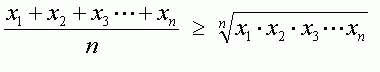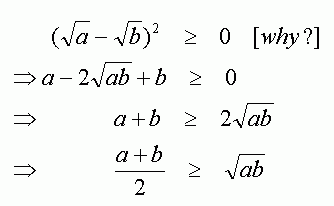SEARCH HOMEMath Central Quandaries & QueriesQuestion from dani, a student: Hi! I was just wondering why the arithmetic mean of sets of numbers is larger than the mean proportional of the same numbers? Thanks! DaniHi Dani,

The proportional mean is, in my experience, more commonly called the geometric mean. So, I think your question is how do we know the following inequality is true (the expression on the left is the arithmetic mean, and the expression on the right is the geometric mean):There are a number of ways to prove this; which is easiest would depend on your background. One of the key ideas is that the average of two numbers is larger than the square root of the product of the numbers:There is a Wikipedia entry that outlines some of the proofs:
Inequality of arithmetic and geometric means

-HaleyMath Central is supported by the University of Regina and The Pacific Institute for the Mathematical Sciences.